Blog

## Derivation of rate law describing binding of surface molecules in immunological synapse

Oleg Demin
April 18, 2022

Introduction

One of the ways of immune cells interaction is formation of Immunological Synapse (IS). In the IS surface molecules (SM) located on the surface of different cells are able to bind each other and elicit stimulating (or inhibitory) signaling leading to modulation of cell dynamics processes such as cell proliferation, cytokine production, cytotoxicity etc. To model SM binding in IS it is important to have an expression of the rate law of the process in terms of variables which have clear biological meaning and can be directly measured or estimated from the available experimental data.

In this study we will derive the rate law of SM binding in IS expressed in terms of

• total number of free surface molecules per cell and complex of surface molecules per cell
• fractions of surface of IS for each of interacting cells
• 2-dimensional (2D) equilibrium dissociation constant

Initial model of binding of SM in IS

Let us consider IS of 2 cells. First cell carries ligand (L) and second cell expresses receptor (R) on its surfaces. Fig. 1 represents scheme of the model describing

• synthesis and degradation of R and L at the surface of corresponding cells
• distribution of R and L between IS and surface of the cell not involved in IS formation. We will use designation “nis” (non-immunological synapse) for such type surface or SM located in it.
• binding of R and L in IS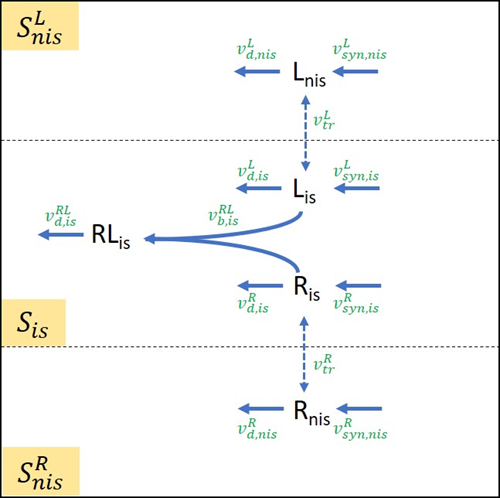Fig. 1. Scheme of the model describing synthesis, degradation, distribution and binding of surface molecules L and R in 2 cells forming IS. Here we use following designations: “is” – subscript referring to IS, “nis” – subscript referring to surface of the cell not involved in IS formation;  is surfaces area (expressed in um2) of IS, ,  are surface areas of cell expressing L and R correspondently (expressed in um2) which are NOT involved in IS formation; Lis, Lnis are 2D concentrations of free L in IS and NIS compartments (expressed in molecules/um2); Ris, Rnis are 2D concentrations of free R in IS and NIS compartments (expressed in molecules/um2); RLis is 2D concentration of RL complex in IS (expressed in molecules/um2);  are rate laws of corresponding processes (expressed in molecules/hour) where , “syn”, “d”, “tr” and “b” stands for synthesis, degradation, transition and binding, correspondently.

ODE system corresponding to scheme shown at Fig. 1 can be written down in following way: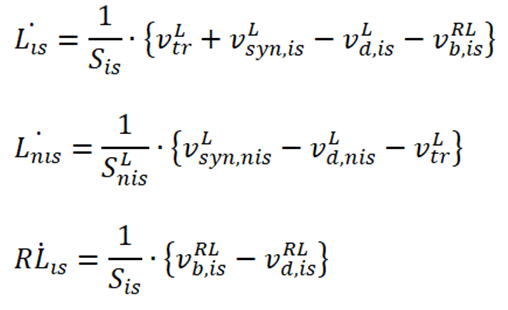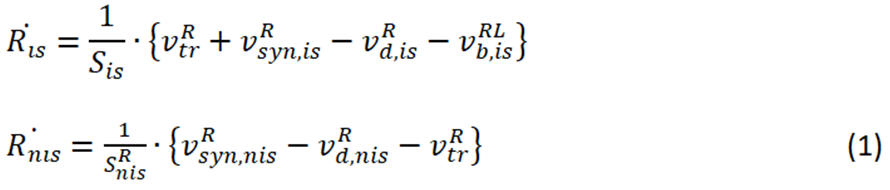Surface areas of IS and NIS compartments obeys following equations representing conservation laws of surface area for each of the cell involved in IS formation: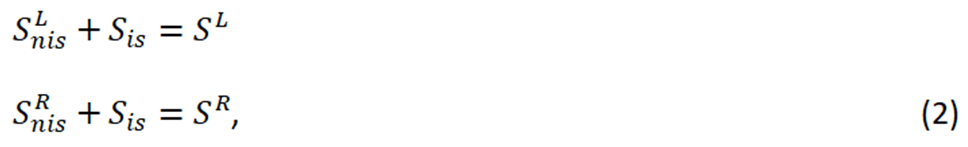Here,  and  are total surface areas of cells expressing L and R, correspondently.

Rate laws of right-hand side of Eqs (1) can be expressed in terms of variables in following manner: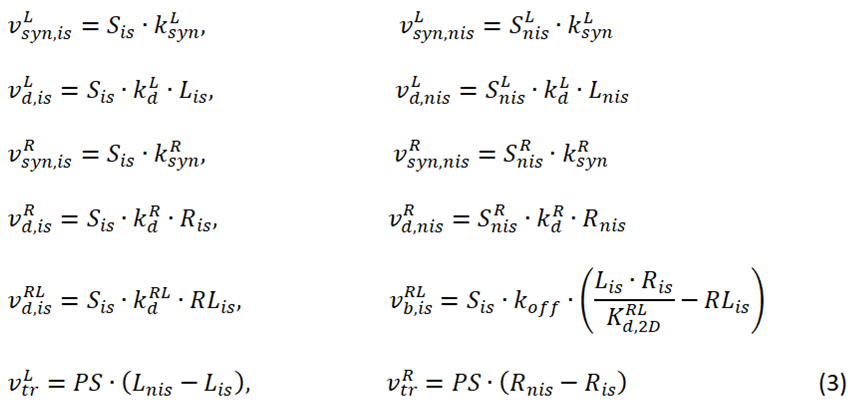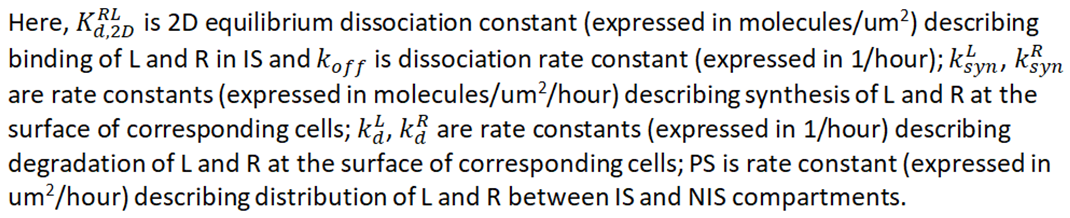Derivation of rate law of binding of SM in IS in terms of total number of SM per cell

Equation for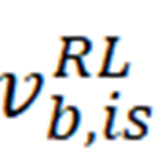(see formular (3)) describes rate law of L and R binding in IS in terms of free concentration of L, R and concentration of their complex RL in IS. Let us try to transform the equation in such a way to express free concentration in IS in terms of total number of surface molecules per cell. For the purposes we will use an assumption that transitions of free L and R between IS and NIS compartments are fast processes, i.e. the processes can be considered at quasi equilibrium.

Let us introduce following new variables of our model: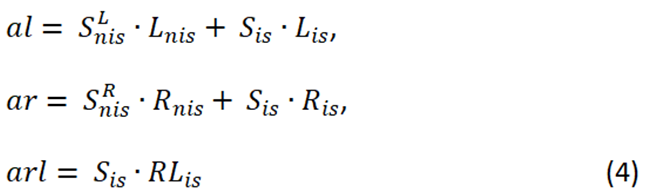These variables represent number of free molecules of L per cell expressing L (al), number of free molecules of R per cell expressing R (ar) and number of complexes RL per IS (arl) or per each of the cell forming IS.

Multiplying 1st and 2nd equations of ODE system (1) by Sis and SLnis and summing them up, multiplying 4th and 5th equations of ODE system (1) by Sis and SLnis and summing them up, multiplying 3rd equations of ODE system (1) by Sis and tending PS to infinity (on the basis of the assumption of quasi-equilibrium for transition of free forms of L and R between different compartments), one obtains following ODE system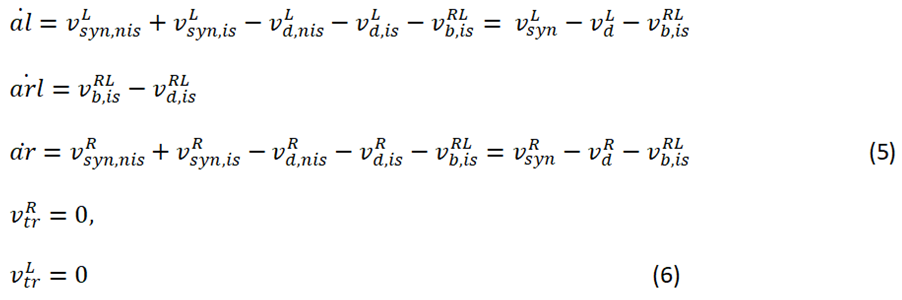Solving equations (4) and (6) together we will express old variables (Lis , Lnis , Ris , Rnis , RLis ) in terms of new variables (al, ar, arl):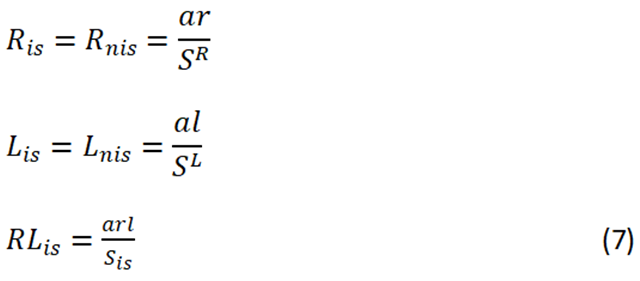Using equations (7) we can rewrite rate law describing binding of L and R in IS in following manner: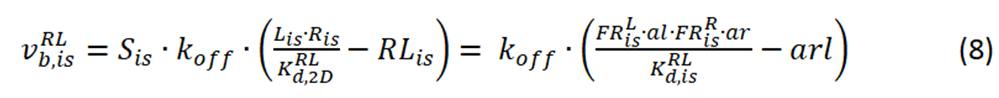Here, FRLis and FRRis represent fractions of cell surface corresponding to IS in cells expressing L and R correspondently,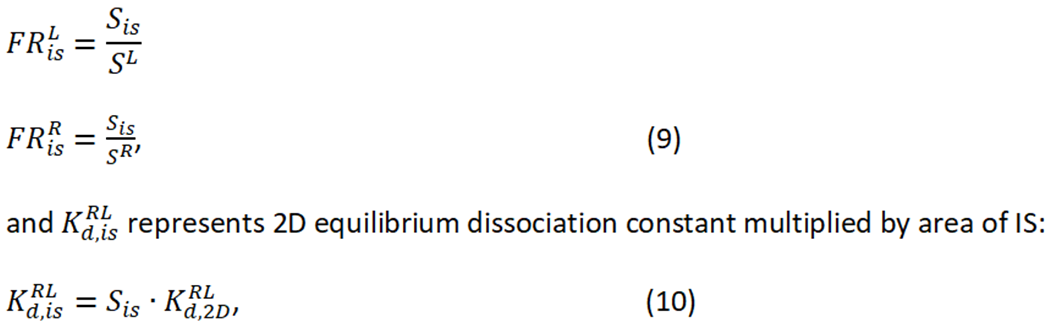Other rate laws of right-hand sides of (5) looks as follows: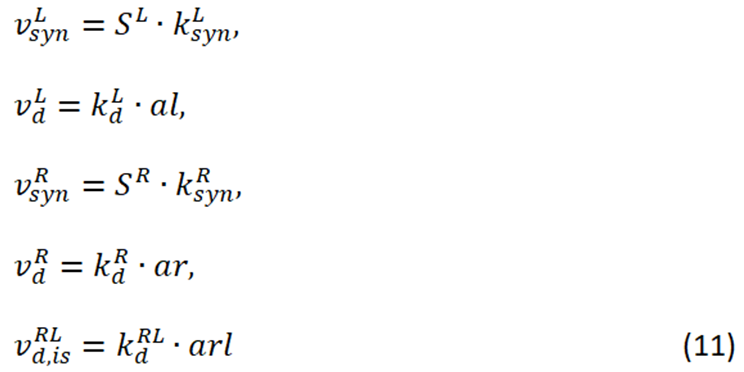ODE system (5) with rate laws (8)- (11) corresponds scheme shown in the Fig. 2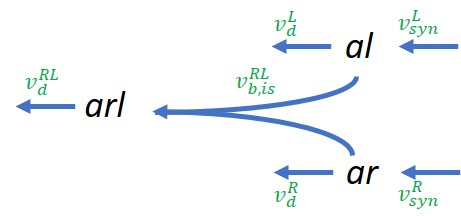Fig. 2. Scheme of reduced model describing binding of surface molecules in IS